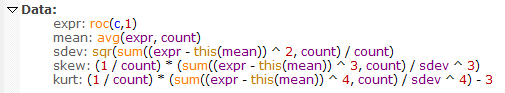Navigation: Realtest Script Language > Syntax Element Details > Skewness

Category

Multi-Bar Functions

Description

Statistical measure of the lopsidedness of a distribution of values

Syntax

Skewness(expr, count)

Parameters

expr - data series formula

count - lookback period

Notes

Skewness is calculated in the same way that Excel calculates the SKEW.P function, which is as if the set of count values is the entire population.

The specific formula used is shown below, in the "skew" item:This also illustrates how these statistical functions could be calculated in the Data Section of a script, though since they're provided built-in, there's no reason to do so.

This function supports ultra-fast one-pass calculation when used in the Data Section with a non-variable count.